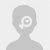# C E ' H A L O

Thailand
On Pinkoi since 2015-03-19
Listings
137
Items sold
143
Followers
1,681ce-ha-lo
Response rate
95%
Response time
1-3 days
Avg. time to ship
Over 7 days
• Items137
• Shop Story
• Return & Exchange Policy

More
C E ' H A L O

US\$ 90.09

C E ' H A L O

US\$ 90.09

C E ' H A L O

US\$ 12.02

C E ' H A L O

US\$ 28.45

C E ' H A L O

US\$ 28.45

C E ' H A L O

US\$ 28.45

C E ' H A L O

US\$ 28.45

C E ' H A L O

US\$ 12.65

C E ' H A L O

US\$ 44.26

C E ' H A L O

US\$ 53.74

C E ' H A L O

US\$ 20.55

C E ' H A L O

US\$ 14.23

C E ' H A L O

US\$ 24.98

C E ' H A L O

US\$ 24.98

C E ' H A L O

US\$ 24.98

C E ' H A L O

US\$ 24.98

C E ' H A L O

US\$ 6.33

C E ' H A L O

US\$ 12.65

C E ' H A L O

US\$ 9.01

C E ' H A L O

US\$ 9.01

C E ' H A L O

US\$ 9.01

C E ' H A L O

US\$ 9.01

C E ' H A L O

US\$ 9.01

C E ' H A L O

US\$ 9.01

C E ' H A L O

US\$ 9.01

C E ' H A L O

US\$ 6.33

C E ' H A L O

US\$ 24.98

C E ' H A L O

US\$ 24.98

C E ' H A L O

US\$ 24.98

C E ' H A L O

US\$ 12.65

C E ' H A L O

US\$ 12.65

C E ' H A L O

US\$ 12.65

C E ' H A L O

US\$ 12.65

C E ' H A L O

US\$ 12.65

C E ' H A L O

US\$ 12.65

C E ' H A L O

US\$ 12.65

C E ' H A L O

US\$ 20.55

C E ' H A L O

US\$ 26.87

C E ' H A L O

US\$ 6.33

C E ' H A L O

US\$ 9.01

C E ' H A L O

US\$ 9.01

C E ' H A L O

US\$ 9.01

C E ' H A L O

US\$ 9.01

C E ' H A L O

US\$ 9.01

C E ' H A L O

US\$ 9.01

C E ' H A L O

US\$ 9.01

C E ' H A L O

US\$ 24.98

C E ' H A L O

US\$ 21.82

C E ' H A L O

US\$ 12.65

C E ' H A L O

US\$ 12.65

C E ' H A L O

US\$ 12.65

C E ' H A L O

US\$ 12.65

C E ' H A L O

US\$ 12.65

C E ' H A L O

US\$ 12.65

C E ' H A L O

US\$ 24.98

C E ' H A L O

US\$ 21.82

C E ' H A L O

US\$ 24.98

C E ' H A L O

US\$ 44.26

C E ' H A L O

US\$ 6.33

C E ' H A L O

US\$ 9.01